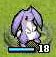星之一角

What have you found for these years?

2008-07-29

如果將字母 A 到 Z 分別編上 1 到 26 的分數

19+3+15+16+9+3+19+9+12+9+3+15+22+15+12+3+1+14+15+3+15+14+9+15+19+9+19=560）

http://en.wikipedia.org/wiki/Pneumonoultramicroscopicsilicovolcanoconiosis

2 retries: